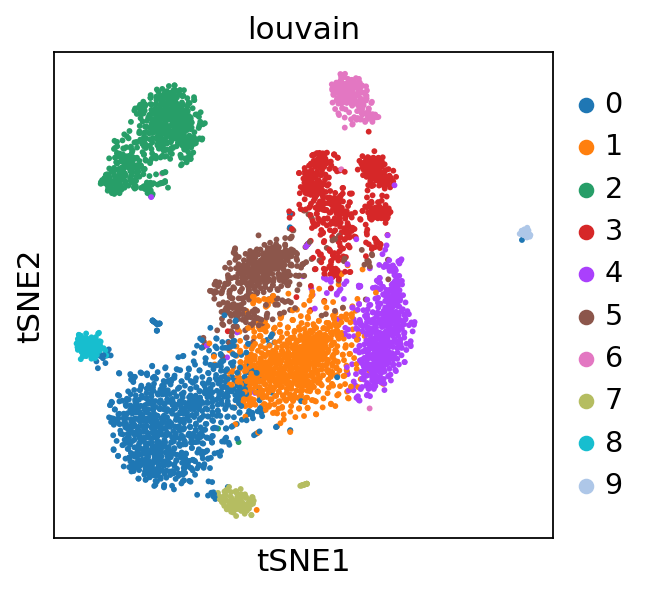This notebook shows how to do gene regulatory analysis for scRNA-Seq dataset using SCENIC. The example data used in this notebook is the PBMC4k dataset downloaded from 10xGenomics

SCENIC package allows users to characterize the single-cell gene regulatory network interference and cluster cell by set of regulons. Below is the main steps in SCENIC workflow:

1. Building the gene regulatory network (GRN):

a. Identify potential targets for each TF based on co-expression.

• Filtering the expression matrix and running GENIE3/GRNBoost.
• Formatting the targets from GENIE3/GRNBoost into co-expression modules.

b. Select potential direct-binding targets (regulons) based on DNA-motif analysis (RcisTarget: TF motif analysis)

1. Identify cell states and their regulators:

c. Analyzing the network activity in each individual cell (AUCell)

• Scoring regulons in the cells (calculate AUC)
• Optional: Convert the network activity into ON/OFF (binary activity matrix)

d. Identify stable cell states based on their gene regulatory network activity (cell clustering) and exploring the results.

In :
import os, sys, glob, pickle
import operator as op

import pandas as pd
import seaborn as sns
import numpy as np
import scanpy as sc
import loompy as lp
import matplotlib as mpl
import matplotlib.pyplot as plt
from matplotlib.pyplot import rc_context

from pyscenic.transform import df2regulons
from pyscenic.binarization import binarize

from IPython.display import HTML, display


Set some settings for Scanpy about verbosity and the figure size

In :
sc.set_figure_params(dpi=150, fontsize=10, dpi_save=600)
sc.settings.njobs = 16


### Data preprocessing¶

Get the expression data

In [ ]:
os.system('wget https://cf.10xgenomics.com/samples/cell-exp/2.1.0/pbmc4k/pbmc4k_filtered_gene_bc_matrices.tar.gz')

In [ ]:
os.system('tar -xvzf pbmc4k_filtered_gene_bc_matrices.tar.gz')

In :
adata = sc.read_10x_mtx(
'./filtered_gene_bc_matrices/GRCh38/' ,
var_names='gene_symbols',
)


Filter low-quality cells

In :
# simply compute the number of genes per cell (computers 'n_genes' column)

# for each cell compute fraction of counts in mito genes versus all genes

# initial cuts

In :
adata

Out:
View of AnnData object with n_obs × n_vars = 4335 × 33694
obs: 'n_genes', 'percent_mito', 'n_counts'
var: 'gene_ids'

Basic pre-processing

In :
# save a copy of the raw data

# Total-count normalize (library-size correct) to 10,000 reads/cell

# log transform the data.

# identify highly variable genes.

# keep only highly variable genes:

# regress out total counts per cell and the percentage of mitochondrial genes expressed

# scale each gene to unit variance, clip values exceeding SD 10.

In :
adata

Out:
AnnData object with n_obs × n_vars = 4335 × 1775
obs: 'n_genes', 'percent_mito', 'n_counts'
var: 'gene_ids', 'highly_variable', 'means', 'dispersions', 'dispersions_norm', 'mean', 'std'
uns: 'log1p', 'hvg'

We write the basic filtered expression matrix to a loom file. This will be used in the command-line pySCENIC steps

In :
# create basic row and column attributes for the loom file:
row_attrs = {
}
col_attrs = {
}


Perform dimensionality reduction and clustering

In [ ]:
sc.tl.pca(adata, svd_solver='arpack')

# neighborhood graph of cells (determine optimal number of PCs here)

# compute tSNE

# cluster the neighbourhood graph

In :
sc.pl.tsne(adata, color=['louvain'])### Cell type labeling¶

In :
with rc_context({'figure.figsize': (2, 2)}):